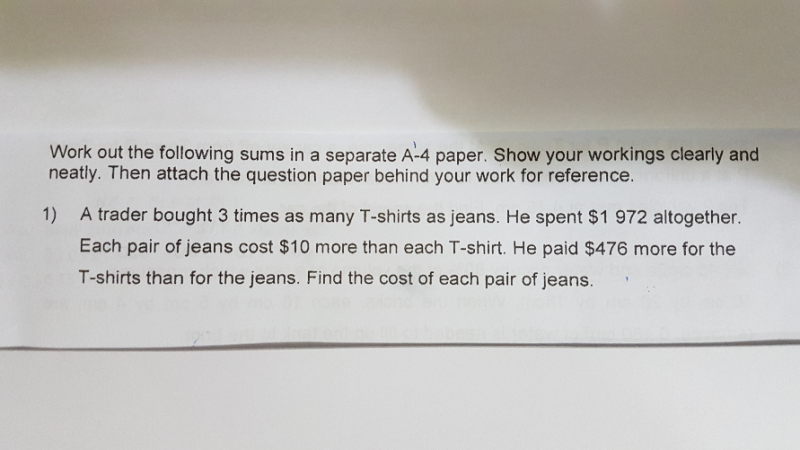# QuestionHi teachers, i need help for this P6 Math question. Thank you.

This is a Quantity Each Product question.

 Quantity Each Poduct Jean 1u 748 T shirt 3u 1224 1972

1972  – 476 = 1496

1496 ÷ 2 = 748

1972 – 748 = 1224

748 / 1u  – 1224/ 3u = 10

2244/ 3u – 1224 / 3u = 10

2244 – 1224 = 30u

30u  = 1020

1u = 1020 ÷ 30 = 34 jeans

748 ÷ 34 = \$22

0 Replies 0 Likes